info@biomedres.us   +1 (720) 414-3554
One Westbrook Corporate Center, Suite 300, Westchester, IL 60154, USA

# Biomedical Journal of Scientific & Technical Research

July, 2019, Volume 20, 1, pp 14806-14808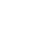Mini Review

Mini Review

# On the Possibility of Detecting Different Kinds of Diseases with the Help of Fixation of Electromagnetic Radiation Intensity of Red Blood Cells

Author Affiliations

Moscow aviation institute (MAI), Russia

Received: July 22, 2019 | Published: July 30, 2019

Corresponding author: Gladkov SO, Moscow aviation institute (MAI), Moscow, Russia

DOI: 10.26717/BJSTR.2019.20.003404

#### Abstract

Based on the general principles of the theory of electromagnetic radiation, bound to charged particles, it is shown that any living biological object emits electromagnetic waves of a certain intensity and power induced by the movement of red blood cells by curves. Noted, a what If for standard electromagnetic radiation take intensity healthy body, the when any violations functional abilities what or internal bodies always can fix local deviation radiated intensity from reference values, such way, install probable place disease.

Keywords: Curvilinear Trajectory; Electromagnetic Radiation; Electric Charge of Erythrocytes; Power of Radiation of Biological Object

#### Mini-Review

The question, which is devoted to the real work, has its rather long history, which dates back to the times of the last century, when closely began to deal with various incomprehensible phenomena such as moving objects without an explicit external Impact. In our recently published work  Was given a detailed mathematical solution to the problem of synchronization of two complanar physical pendulums, in which from a purely physical point of view it was strictly proved that the nature of this interesting phenomenon is purely electromagnetic in nature, and It is connected with the radiating ability of the pendency, and, as it turned out, it is absolutely unimportant whether they are metallic or made of plastic or even wooden.

In order to present the essence of this article we will use the basic principles and ideas of work , and we will represent a living organism, simulating it simply as a physical body within which streams of charged particles (erythrocytes) move. As is known , when the charges are moving with some variable speed v, they create a distance r non-stationary and spatially-heterogeneous electromagnetic field. Next we are will abbreviated characterize it acronym EM. It should be emphasized, however, that due to the far action characters of the EM field, the distance is not limited. Corresponding EM fields are connected with electric potential φ =φ (r,t) and magnetic A = A(r,t) simple linear rations, such as the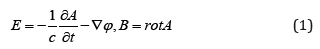where E- is the electric field tension, B- is magnetic induction vector.

In the present case, that is, in the case of moving charges, in a real environment these potentials can be represented by the following symmetrical expressionswhere e- is the particle charge, c- is a speed of light in a vacuum, ε- is the dielectric permeability of the substance, and μ- is magnetic permeability,is a distance from charge to observation point, r- is point of observation, is a trajectory of the moving charge. If you introduce the average concentration of red blood cells n, by analogy with the work  for the respective potentials will have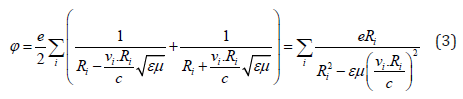where the number characterizes the appropriate organ on which the blood flow moves.

Rations (3) tell us that the intensity of radiation, which is known , proportional to the square of the derivative of the velocity of the charged particle in time, should be characterized by an electric field of radiation generated by a moving charge, which according to (1) can be found asand according to (3) will beTherefore, the total intensity of the radiation will amount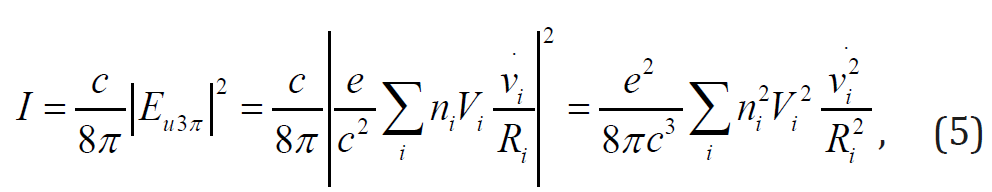Where we believe that by virtue of the independence of diverse terms, only the squares of the corresponding values are left under the sign of the sum (like calculation of intensity at interference of EM waves). By and large this result is easy to understand, if we consider that the movement of blood, say, in the kidneys does not correlate with its movement, for example, in the liver or in the spleen. When the correlation between all organs is taken into account, the expression (5) will no longer be so compact. In this communication we will not be interested in such correlation.

Thus, the total biological power of the whole organism, which is obliged to the movement of red blood cells, should be defined as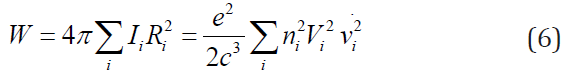To assess the magnitude of the figure, it should be remembered that blood is a hydrodynamic flow on the capillaries and vessels of the body, and therefore can be described within the equation of Navier-Stokes. Indeed, according to for example (3) we have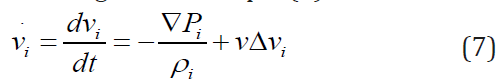Where Pi- is a pressure inside i- body, ρi- is blood density in this i- th body, v- is kinematic viscosity? Ignoring the last term in the right part of the equation (7), we find the connection that interests usSubstituting (8) in the expression (6) for the full power of radiation we get as a result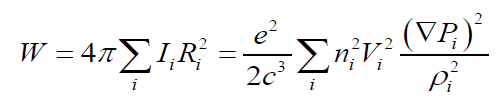Since the same density can be imagined as, ρi=mni where m- is the mass of the local charged area of erythrocytes (that is, a small part of the whole mass of erythrocytes), come to the next remarkable formula, describing the full radiation of the entire bodyNote that the volume in this formula Vi can be considered known, which is not yet possible to assert about the pressure gradient. To estimate this, we use the following general physical considerations. Indeed, because blood flows through each organ, the necessary condition for its movement on it is the differential pressure at the inlet P2i and P1i outlet. This means that if the linear dimension iorgan there Li, the absolute value of the pressure gradient can be presented in the form of the next estimated ratio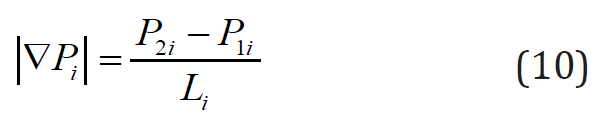If you substitute now (10) in the general formula (9), we find the result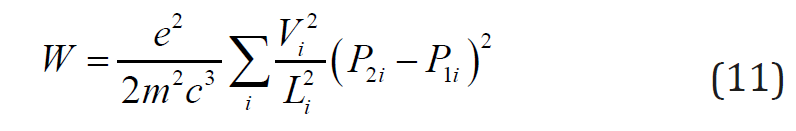It should be said that in order of magnitude the pressure in any of our organs should be roughly equal to that of arterial, which can be denoted as P. The differential pressures at the inlet and outlet should be of the same order, because otherwise the blood would just stand still. This means that for any organ we can assume, first, that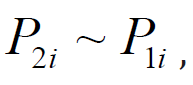and, secondly, to believe that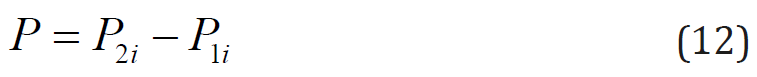Therefore, the expression (11) is even more simplified, and we get the following formula for estimating the power of radiationThe formula (13) responds to the question posed at the beginning of the article on the power of radiation characterizing any living organism, the origin of which is associated only with the radiation, going from red blood cells. According to (13) it can be easily appreciated. As to the general EM background of the biological body, it is characterized as known by the usual thermal radiation, and is described by the formula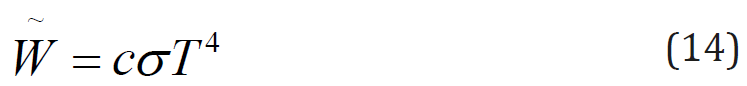where σ- is the Stefan-Boltzmann’s constant, T- is a temperature? It is clear that full radiation should be defined as the sum of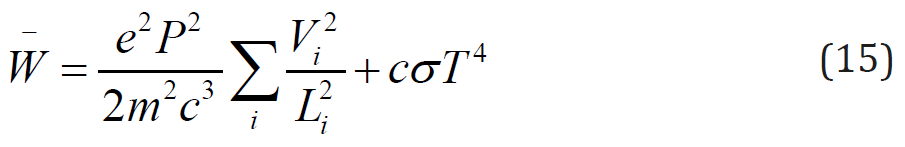To quantify the radiation (13), we need to set the appropriate parameters. ~

We will assume that the charge of erythrocyte is e ~ 1018CTC (this value is taken by us, based only on general physical considerations, because in the reference books on medicine we simply did not find it), and in order of magnitude we put pressure equal P ~ 104 Pa . The mass of the local charged area of red blood cells may be, for example, m ~ 10−15 g Speed of light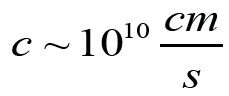The average linear size of each organ, based simply on purely assessment considerations, will be considered the same and we assume that Li ~ 10cm. Finally, the total number of all the main organs of our body we will accept equal to one hundred.

As a result of substitution of the given purely estimation numerical values in the general formula (13) цe immediately find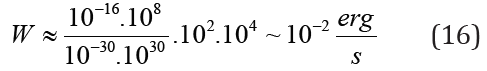If the erg/s is transferred to watts, it will be approximately 10−9Watt. As we can see, the value of radiation power is quite small, but it clearly indicates the possibility of its fixation with the help of EM radiation detectors.

The noted fact allows us to easily estimate EM radiation, which is obliged to the charged particles of an organism which is an inherent property of any biological object.

#### Conclusion

In conclusion of the article we note a number of important points.
1. It is shown that the power of radiation of charged particles of an organism makes a comparatively small amount of order 10−9Watt.
2. At any painful manifestations the power should be less than this reference.
3. The exact value of the radiation power can only be found through the experiment.

Mini Review

# On the Possibility of Detecting Different Kinds of Diseases with the Help of Fixation of Electromagnetic Radiation Intensity of Red Blood Cells

Author Affiliations

Moscow aviation institute (MAI), Russia

Received: July 22, 2019 | Published: July 30, 2019

Corresponding author: Gladkov SO, Moscow aviation institute (MAI), Moscow, Russia

DOI: 10.26717/BJSTR.2019.20.003404

#### Abstract

Based on the general principles of the theory of electromagnetic radiation, bound to charged particles, it is shown that any living biological object emits electromagnetic waves of a certain intensity and power induced by the movement of red blood cells by curves. Noted, a what If for standard electromagnetic radiation take intensity healthy body, the when any violations functional abilities what or internal bodies always can fix local deviation radiated intensity from reference values, such way, install probable place disease.

Keywords: Curvilinear Trajectory; Electromagnetic Radiation; Electric Charge of Erythrocytes; Power of Radiation of Biological Object